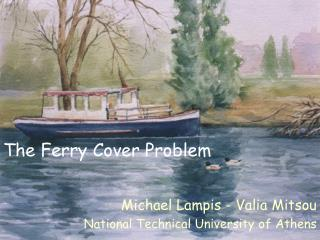DownloadDownload PresentationThe Ferry Cover Problem

# The Ferry Cover Problem

Download Presentation## The Ferry Cover Problem

- - - - - - - - - - - - - - - - - - - - - - - - - - - E N D - - - - - - - - - - - - - - - - - - - - - - - - - - -
##### Presentation Transcript

1. The Ferry Cover Problem Michael Lampis - Valia Mitsou National Technical University of Athens

2. Wolf

3. Goat

4. Cabbage

5. Guard

6. Boat

7. Previous Work • “Propositiones ad acuendos iuvenes”, Alcuin of York, 8th century A.D (in latin). • We propose a generalization of Alcuin’s puzzle

8. Our generalization

9. Our generalization • We seek to transport n items, given their incompatibility graph. • Objective: Minimize the size of the boat • We call this the Ferry Cover Problem

10. OPTFC (G) ≥ OPTVC (G)

11. OPTFC (G) ≥ OPTVC (G)

12. OPTFC (G) ≥ OPTVC (G)

13. OPTFC (G) ≤ OPTVC (G) + 1

14. OPTFC (G) ≤ OPTVC (G) + 1

15. OPTFC (G) ≤ OPTVC (G) + 1

16. OPTFC (G) ≤ OPTVC (G) + 1

17. OPTFC (G) ≤ OPTVC (G) + 1

18. OPTFC (G) ≤ OPTVC (G) + 1

19. OPTFC (G) ≤ OPTVC (G) + 1

20. OPTFC (G) ≤ OPTVC (G) + 1

21. OPTFC (G) ≤ OPTVC (G) + 1

22. OPTFC (G) ≤ OPTVC (G) + 1

23. OPTFC (G) ≤ OPTVC (G) + 1

24. OPTFC (G) ≤ OPTVC (G) + 1

25. The Ferry Cover Problem Lemma: OPTVC (G) ≤ OPTFC (G) ≤ OPTVC (G) + 1 Graphs are divided into two categories: • Type-0, ifOPTFC (G) = OPTVC (G) • Type-1, if OPTFC (G) = OPTVC (G) + 1

26. Hardness and Approximation Results • Ferry Cover is NP and APX-hard (like Vertex Cover [Håstad1997]). • A ρ-approximation algorithm for Vertex Cover yields a (ρ+1/ OPTFC)-approximation algorithm for Ferry Cover.

27. Ferry Cover in other well known graph topologies